# How to Calculate and Solve for General Binomial Distribution | Probability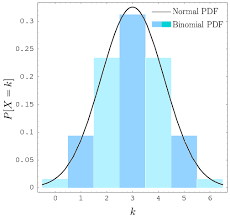The image above represents general binomial distribution.

To compute for general binomial distribution, four essential parameters are needed and these parameters are n, r, q and q.

The formula for calculating general binomial distribution:

P(r successes) = n!(n – r)!r!q(n – r)pr

Where;

P(r successes) = General Binomial Distribution
p = P(A)
q = P(not A)

Let’s solve an example;
Find the general binomial distribution when n is 8, r is 6, p is 1 and q is 0.

This implies that;

n = 8
r = 6
p = 1
q = 0

P(r successes) = n!(n – r)!r!q(n – r)pr
P(6 successes) = 8!(8 – 6)!6!(0)(8 – 6)(1)6
P(6 successes) = 8!2!6!(0)2(1)6
P(6 successes) = 40320(2)(720)(0)2(1)6
P(6 successes) = 403201440(0)2(1)6
P(6 successes) = (28)(0)2(1)6
P(6 successes) = (28)(0)(1)
P(6 successes) = 0

Therefore, the general binomial distribution is 0.

Nickzom Calculator – The Calculator Encyclopedia is capable of calculating the general binomial distribution.

To get the answer and workings of the general binomial distribution using the Nickzom Calculator – The Calculator Encyclopedia. First, you need to obtain the app.

You can get this app via any of these means:

To get access to the professional version via web, you need to register and subscribe for NGN 1,500 per annum to have utter access to all functionalities.
You can also try the demo version via https://www.nickzom.org/calculator

Apple (Paid) – https://itunes.apple.com/us/app/nickzom-calculator/id1331162702?mt=8
Once, you have obtained the calculator encyclopedia app, proceed to the Calculator Map, then click on Probability under Mathematics.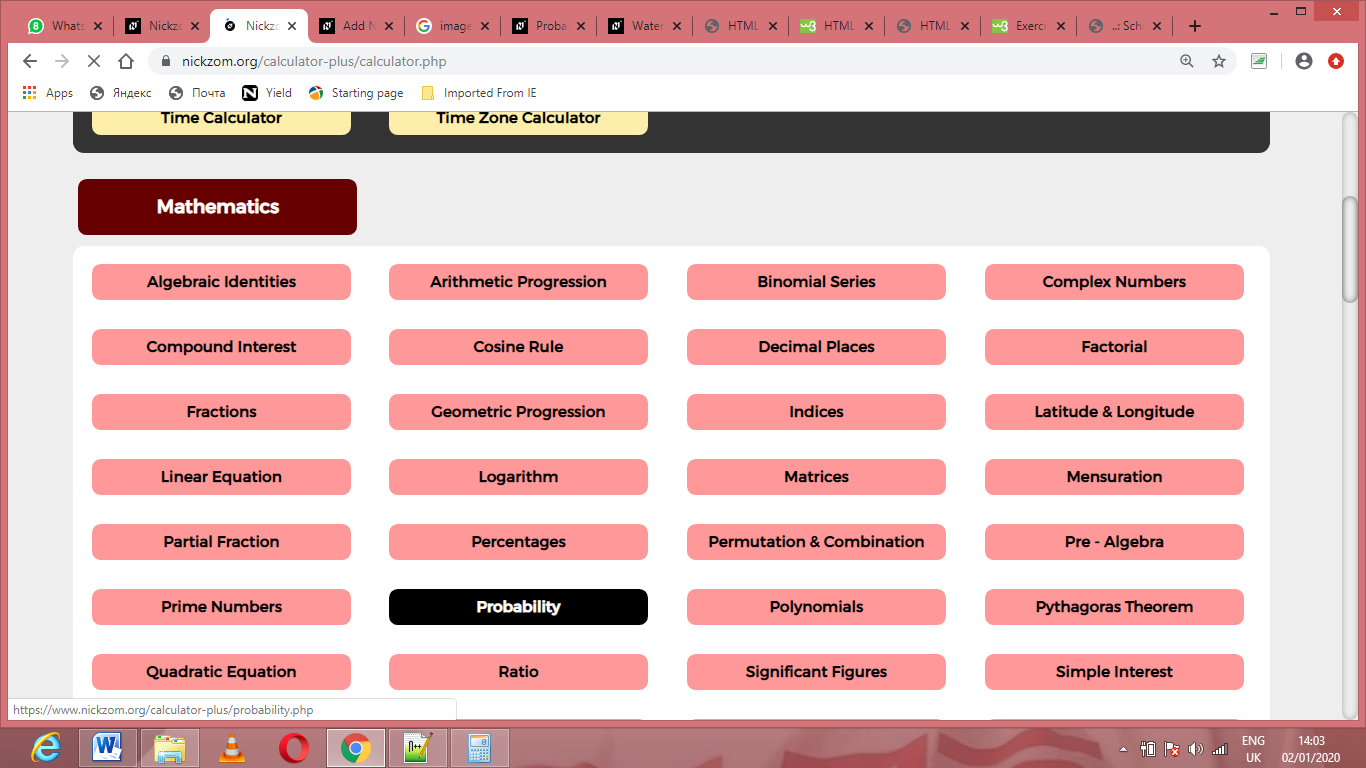Now, Click on General Binomial Distribution under Probability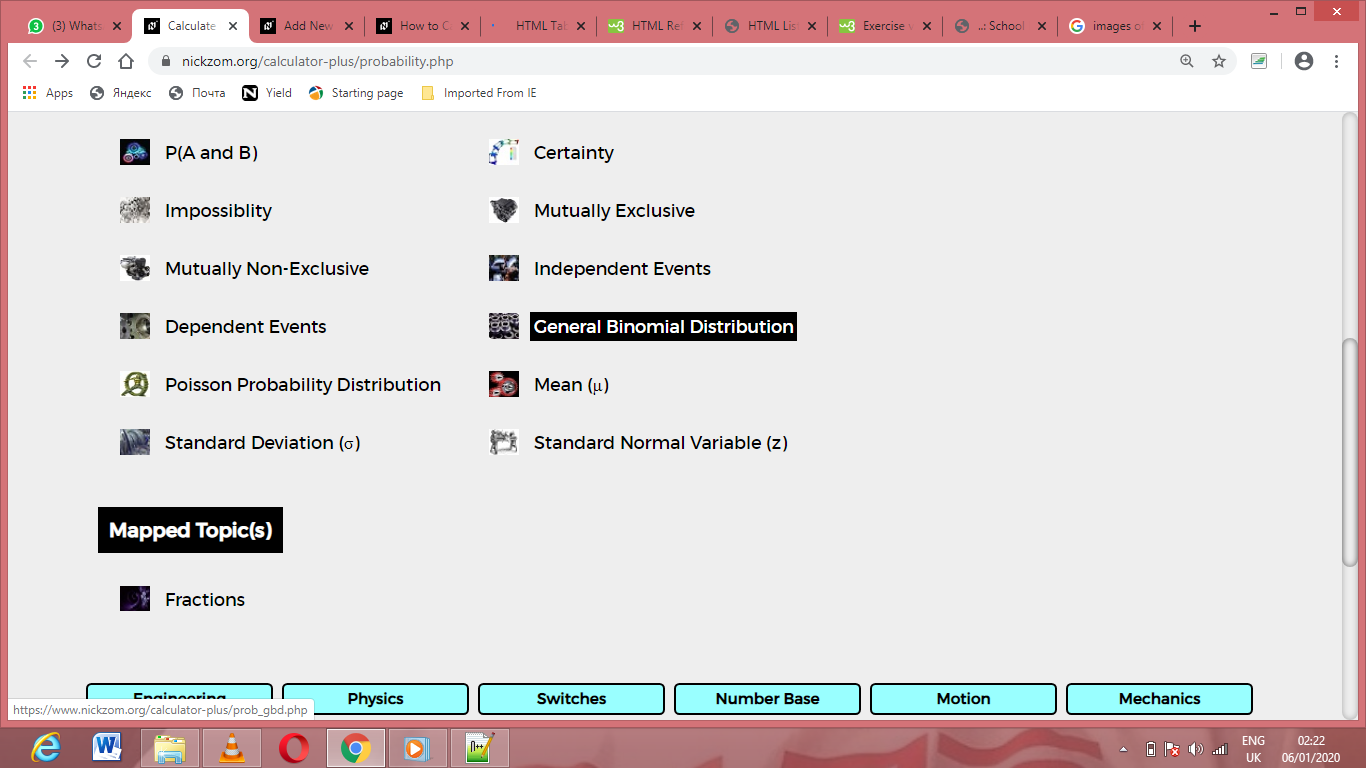The screenshot below displays the page or activity to enter your values, to get the answer for the general binomial distribution according to the respective parameters which are the n, r, p and q.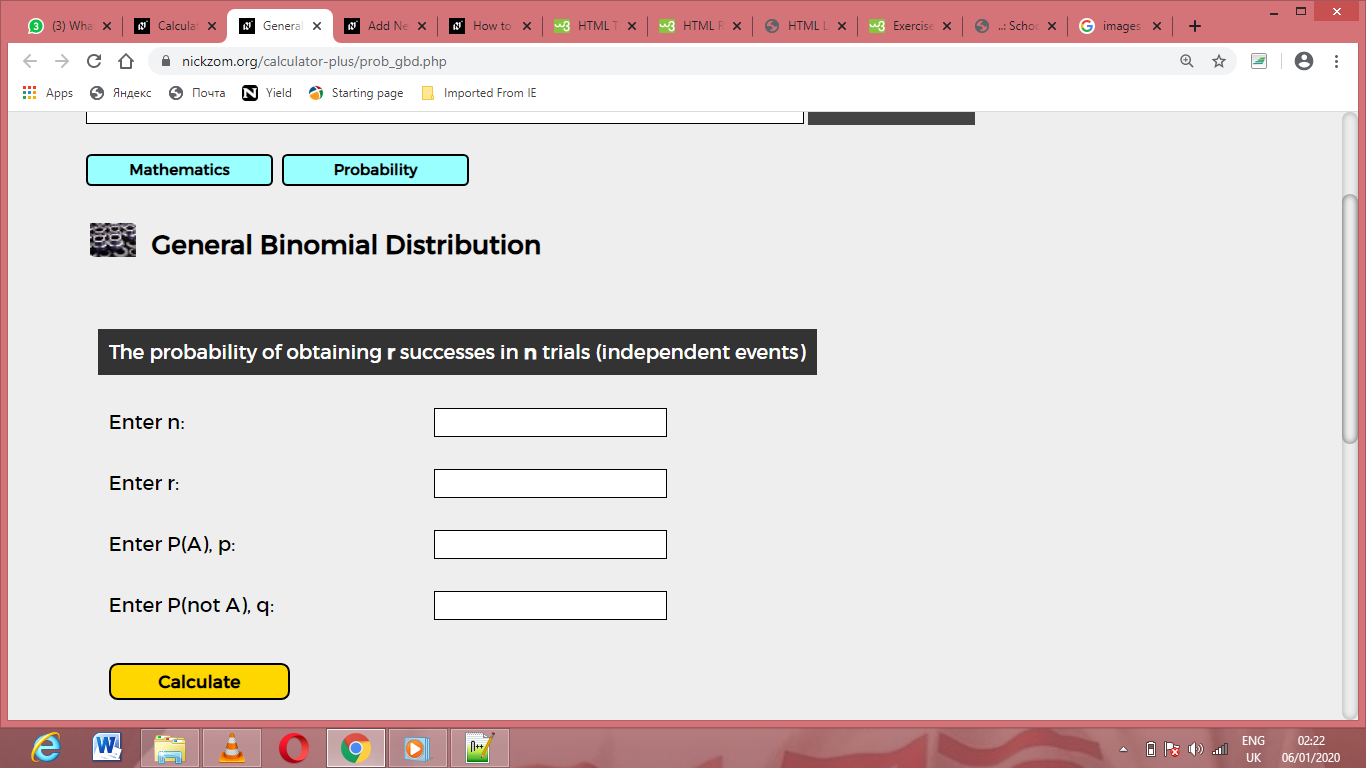Now, enter the values appropriately and accordingly for the parameters as required by the n is 8, r is 6, p is 1 and q is 0.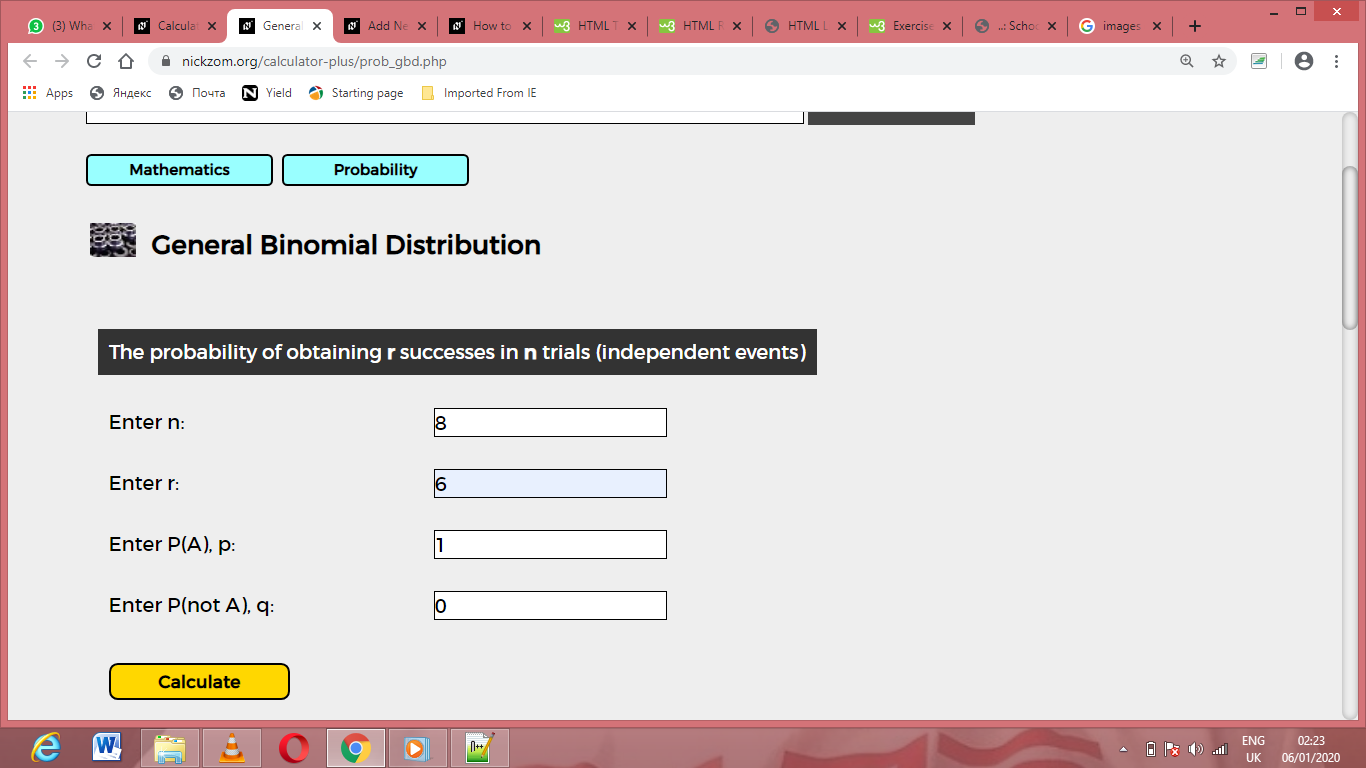Finally, Click on CalculateAs you can see from the screenshot above, Nickzom Calculator– The Calculator Encyclopedia solves for the general binomial distribution and presents the formula, workings and steps too.